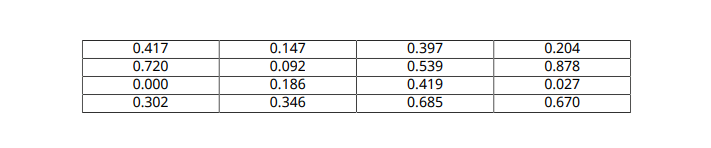# Format Numbers in Tables

This example shows how to format numbers in a table that is generated by a report generation program. The example creates a table of uniformly distributed random numbers that have a precision of three digits after the decimal point.The numbers are formatted by using an `mlreportgen.dom.NumberFormat` style object with the table.

### Generate Random Numbers

Generate a 4-by-4 array of random numbers. Initialize the random number generator using a seed of `1`, so that each time the example runs, `rand` produces the same numbers.

```format long rng("default"); rng(1); randNumbers = rand(4)```

The numbers display with a precision of 15 digits after the decimal point.

### Create a Document

Import the DOM package so that you do not have to use long, fully-qualified class names.

`import mlreportgen.dom.*`

Create a PDF document. To create a Microsoft® Word, HTML, or single-file HTML document, change `"pdf"` to `"docx"`, `"html"`, or `"html-file"`, respectively.

`d = Document("randomnumbers","pdf");`

### Create a Table

Create a DOM table from the array of random numbers.

`t = Table(randNumbers);`

### Specify the Table Style

Specify that numbers in the table have a precision of 3 digits after the decimal point by using an `mlreportgen.dom.NumberFormat` object. Specify the table width, border, and column and row separators.

```t.Style = [t.Style {NumberFormat("%1.3f"),... Width("100%"),... Border("solid"),... ColSep("solid"),... RowSep("solid")}];```

Center the table entries in the table cells.

`t.TableEntriesHAlign = "center";`

### Generate the Report

Append the table to the document. Close and view the document.

```append(d,t); close(d); rptview(d);```